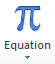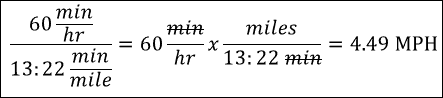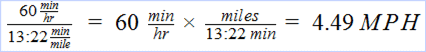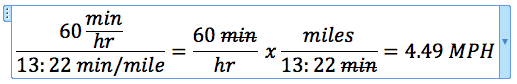# Equation Editors in Excel 2010 and Word 2011The Equation Editor is new in Excel 2010 and designed to create a mathematical equation as a graphical object. I didn’t find it in Excel 2011 for Mac, but ran across an article showing they put it inside Word 2011. How very strange.

Here I’ll review the Excel 2010 version of Equation Editor first, then briefly compare Mathematical Equations in Google Docs, and come back to the Word 2011 version of Equation Editor.

## Excel 2010 Equation Editor

The Equation Editor in Excel 2010 is difficult to navigate at first. The second time I tried creating an equation it got slightly easier, and the third time with the same equation (shown below) I seemed to almost get the hang of it.The trick I learned was to just type the equation in and let the Editor do its thing in rearranging what you’re typing. On the Equation Tools Ribbon are two helpful selections: Professional and Linear (shown below). Playing around with these helped me understand how the Editor can manipulate simple equations.

Having said that, advanced equations will take some time to figure out, but this editor should handle most anything you can throw at it.

## Entering an Equation

To enter an equation with the Equation Editor go to the Insert tab on the Ribbon, and click Equation. This gives you a text box with the words “Type equation here.” You’ll also notice two additional menu tabs on the Ribbon: Equation Tools, which becomes active, and Drawing Tools.

There are varied Symbols readily available for insertion on the Equation Tools Ribbon tab (shown above). And several drop-down menu items that expand to show even more symbols for things like: Fraction, Script, Radical, Integral, Large Operator, Bracket, Function, Accent, Limit and Log, Operator, and Matrix. (Shown below)

Clicking the Equation drop-down arrow will reveal several predefined equations that can be inserted as starting point for your equation. This how I started my first equation, which did me no favors. I fared much better just typing in the formula and letting the Equation Editor move things around.

### One Annoyance

One of the problems with the Equation Editor is that if you stray to far outside the equation, yet still inside the text box, the Equation Tools tab disappears and you’re stuck with the Drawing Tools tab only. Maddening!

I looked at the new Mathematical Equations in Google Docs and it seemed easier to learn, but is not nearly as powerful as the Equation Editor. For example, the equation I created above was done in a Google Docs document with Mathematical Equations. I wasn’t able to do a strikethrough for the min text, like this: min.And there wasn’t a good way to export this object out of Google Docs.

## Equation Editor in Word 2011 for Mac

The Equation Editor in Word 2011 for Mac looks to be identical to the one in Excel 2010. You start an equation from the Document Elements tab on the Ribbon, by clicking Equation or the drop-down menu arrow and selecting a built-in equation.

The Equation Tools tab immediately becomes visible and active after starting an equation. I created the same formula with relative ease.I kind of like the feel of this Word 2011 version better than what’s in Excel 2010. Go figure.## 17 thoughts on “Equation Editors in Excel 2010 and Word 2011”

1.Eric

I’m not so sure that the equation editor is all so new… I’ve been using Microsoft Equation 3.0 as part of the 2003 Office Pack at work for quite a while. I haven’t started to use 2010 yet, so I can’t fully compare.

Under 2003 Office products, choose Object from the Insert menu, and then scroll down to the Microsoft Equation 3.0 object. If it isn’t installed, search on “equation” in Help and follow the directions under the entry for “Insert an equation” for how to install Microsoft Equation 3.0.

It isn’t the greatest product, but it works, and the stuff you have here about 2010 looks very familiar…

1.Gregory

I see what your talking about in Excel 2003. Didn’t know that Microsoft Equation 3.0 even existed, but it also shows up in Excel 2007 and Excel 2010 as the same thing, which is to say an embed object.

The Equation Editor in Excel 2010 is an altogether new offering (my reference here is Excel 2010 Bible) that inserts a text box, not an embedded object, and has some very advanced toolbar features for entering symbols and such.

Thanks for pointing out the Microsoft Equation 3.0 feature for entering equations in older versions of Excel.

2.Patrick

Compatibility between Office programs seems lacking in 2010. I think Microsoft needs to get their act together. For instance:

If you copy and paste the equation object from Word to Excel, you only get a picture object.

If you copy and paste the equation from inside the object from Word to Excel, you get an editable equation, but some information may be lost (for instance if you inserted a symbol from the symbol editor instead of from the equation editor it will be lost).

If you copy and paste the entire equation object from Excel to Word, you still retain the equation, but it has an extra text box surrounding it.

If you copy and paste just the equation from inside the equation object from Excel to Word, the equation appears the same as if you created it in Word.

3.Wayne Davis

I have clicked on the pi symbol in the documents element tab of Word 2011, but the equation editor ribbon never appears. Frustrating.

1.Gregory

Don’t know what to tell you here, unless maybe to check the Equation preferences or Ribbon preferences (in Word Preferences). Maybe there’s something turned off that should be on.

4.seyhan

How to add/insert equation numbers in office for mac 2011?

1.Gregory

I looks like Microsoft Word 2011 is the only program in Office 2011 (Mac) that has the Equation Editor. You start an equation from the Document Elements tab on the Ribbon, by clicking Equation or the drop-down menu arrow and selecting a built-in equation.

5.Doug

It is a giant mess and chore to create any equations at all using this cumbersome system, but after doing so it would be very helpful to have them available for later use. Is there an alternative to this editor that allows easier equation creation? For example, the troff editor allows you to type equation descriptions into an ascii file that is read by the editor. Is there a comparable souce file that can be accessed to insert equations? Or to save the ones that are created?

1.Gregory

No source file available to insert equations. I suppose you could create an Word/Excel file that holds equations that you create, but they would only be available to Microsoft Office programs. This is about as good as it gets with Microsoft Office.

6.Jennifer

My problem with the new equation editor is that they got rid of all the shortcut keys. I used to be able to use alt+f for fractions, ctrl+l for subscripts etc – now I have to use the toolbars. It really slows down the equation writing.

7.Grant

How does one initiate a new line? When I hit enter (or control-enter, or shift-enter, or alt-enter for that matter) it leaves the equation box. I just want to solve the problem in the same equation box, even if it takes multiple lines.

1.Gregory

In the Word 2011 version I use the Fraction structure, which gives a new line. You can then click the drop-down and make sure to pick Change to Display (instead of inline).

8.Grant

How does one enter new lines while staying in the the same equation box. in the 2003 version, you hit “enter” and it went to a new line in the same equation. Do that here, and it leaves the equation box.

1.Gregory

In Word 2011, I use the fraction structure and then take my mouse to click to the bottom box (or top box). Make sure you are in the Display mode by clicking the drop-down button on the Fraction structure and choose Change to Display (if you see Change to inline you are in Display mode).